# Python3 与 NetCore 基础语法对比（Function专栏）

# 定义一个空函数：（不写pass就报错了）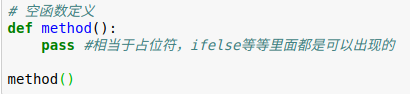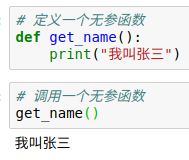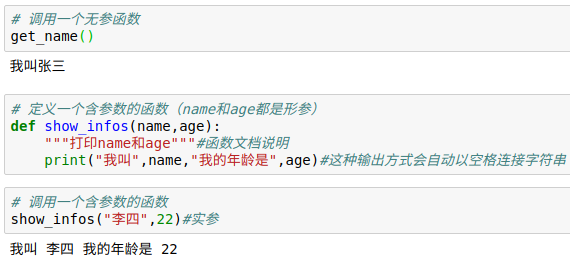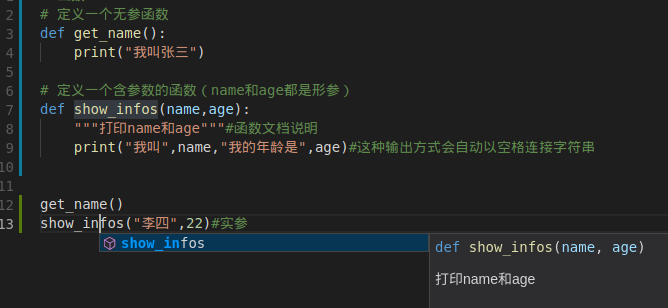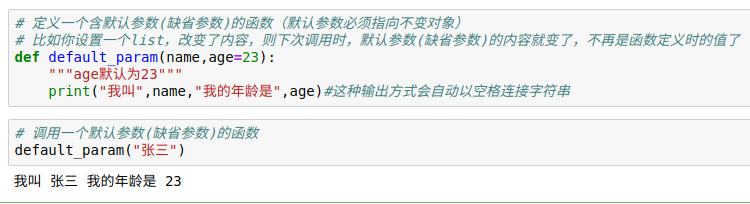# 定义有返回值的函数# 定义含有多个返回值的函数（利用了元组）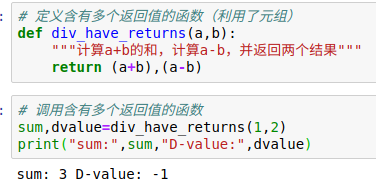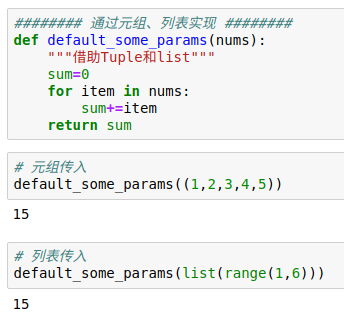# 定义一个可变参数的函数(参数名字一般都是*args)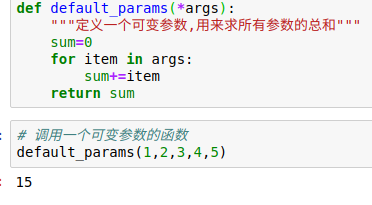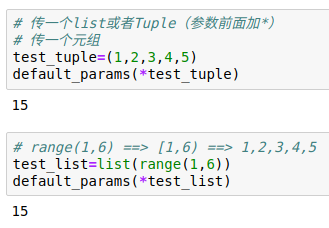# 定义含关键字参数的函数def default_kv_params(name,age=23,**kv):

# 可变参数允许你传入0个或任意个参数，这些可变参数在函数调用时自动组装为一个tuple
# 关键字参数允许你传入0个或任意个含key-value的参数，自动组装为一个dict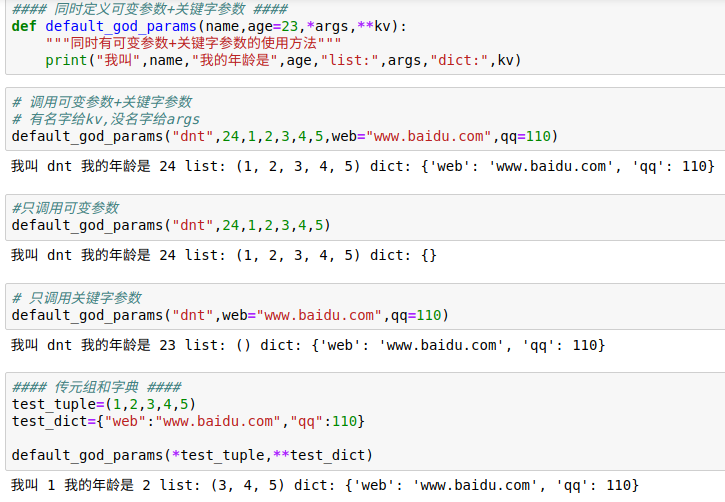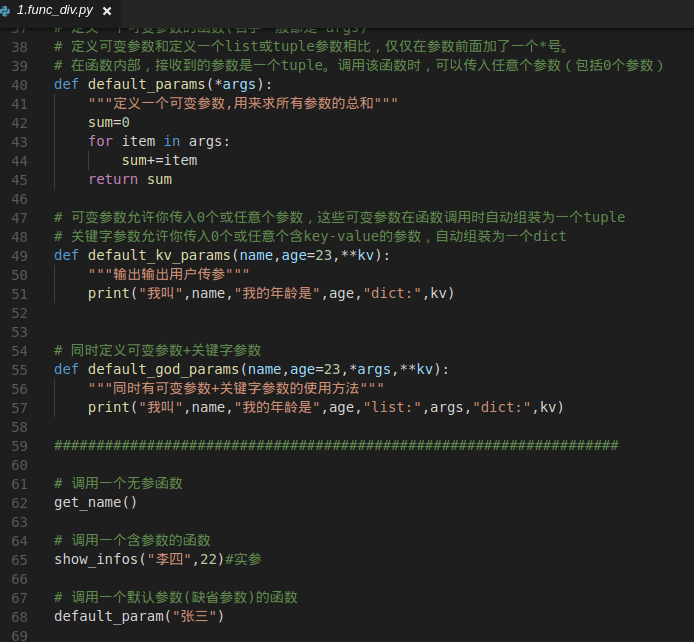NetCore：（C#基础语法大家都很熟了，我贴一个注释的Code即可）
using System;
using System.Collections.Generic;

namespace _6func
{
class Program
{
static void Main(string[] args)
{
#region Base
// # 定义一个空函数：
Method();
// # 定义一个无参函数
GetName();
// # 定义一个含参函数
ShowInfos("李四", 22);
// # 定义一个含默认参数(缺省参数)的函数
DefaultParam("张三");
// # 定义有返回值的函数
int result = DivHaveReturn(1, 2);
Console.WriteLine($"计算结果为{result}"); #endregion // # 定义含有多个返回值的函数（利用了元组） var (sum, dvalue) = DivHaveReturns(1, 2); Console.WriteLine($"sum:{sum},D-value:{dvalue}");

// 传多个参数系列：
// 引用传递（通过元组、列表实现）：扩展有可变类型和不可变类型作为形参的对比
var list = new List<int>() { 1, 2, 3, 4, 5 };
Console.WriteLine(DefaultSomeParams(list));
Console.WriteLine(list.Count);//这就是引用传递的证明

// # 定义一个可变参数的函数(参数名字一般都是*args)
Console.WriteLine(DefaultParams(1, 2, 3, 4, 5));

// # 定义含关键字参数的函数 直接传Dict
}

#region base
/// <summary>
/// 定义一个空函数
/// </summary>
private static void Method()
{

}
/// <summary>
/// 定义一个无参函数
/// </summary>
// private static void GetName()
// {
//     Console.WriteLine("你好");
// }
//简写
private static void GetName() => Console.WriteLine("你好");

/// <summary>
/// 定义一个含参数的函数
/// </summary>
/// <param name="name">名字</param>
/// <param name="age">年龄</param>
// private static void ShowInfos(string name, int age)
// {
//     Console.WriteLine($"我叫{name} 我的年龄是{age}"); // } //简写 private static void ShowInfos(string name, int age) => Console.WriteLine($"我叫{name} 我的年龄是{age}");

/// <summary>
/// 定义一个含缺省参数的函数
/// </summary>
/// <param name="name">名字</param>
/// <param name="age">年龄默认23</param>
// private static void DefaultParam(string name, int age = 23)
// {
//     Console.WriteLine($"我叫{name} 我的年龄是{age}"); // } //简写 private static void DefaultParam(string name, int age = 23) => Console.WriteLine($"我叫{name} 我的年龄是{age}");

/// <summary>
/// 定义一个有返回值的函数（计算a+b，返回计算结果）
/// </summary>
/// <param name="a">num1</param>
/// <param name="b">num2</param>
// private static int DivHaveReturn(int a, int b)
// {
//     return a + b;
// }
//简写
private static int DivHaveReturn(int a, int b) => a + b;
#endregion

/// <summary>
/// 定义含有多个返回值的函数（利用了元组）
/// 计算a+b的和，计算a-b，并返回两个结果
/// </summary>
/// <param name="a"></param>
/// <param name="b"></param>
/// <returns></returns>
// private static (int sum,int dValue) DivHaveReturns(int a, int b)
// {
//     return ((a+b),(a-b));
// }
//简写
private static (int sum, int dValue) DivHaveReturns(int a, int b) => ((a + b), (a - b));

#region 传入多个参数系列
/// <summary>
/// 利用列表实现,引用传递之类的C#还有ref和out，这边就不说了
/// </summary>
/// <param name="nums"></param>
/// <returns></returns>
private static int DefaultSomeParams(List<int> numList)
{
int sum = 0;
foreach (var item in numList)
{
sum += item;
}
numList.Clear();
return sum;
}
/// <summary>
/// 定义一个可变参数的函数
/// </summary>
/// <param name="args"></param>
/// <returns></returns>
private static int DefaultParams(params int[] args)
{
int sum = 0;
foreach (var item in args)
{
sum += item;
}
return sum;
}
#endregion
}
}

1.递归函数:函数递归调用其实就是自己调用自己，关键点只要考虑什么时候跳出即可(没有跳出就是死循环)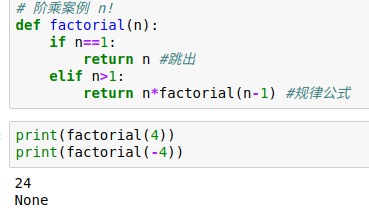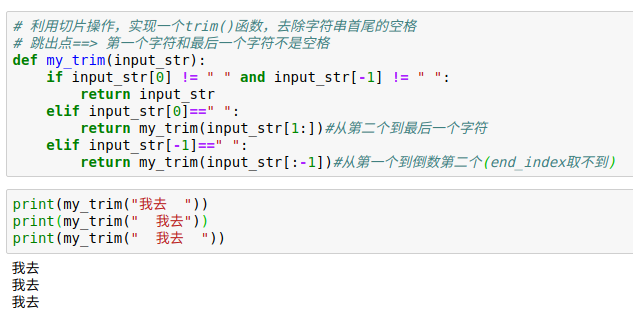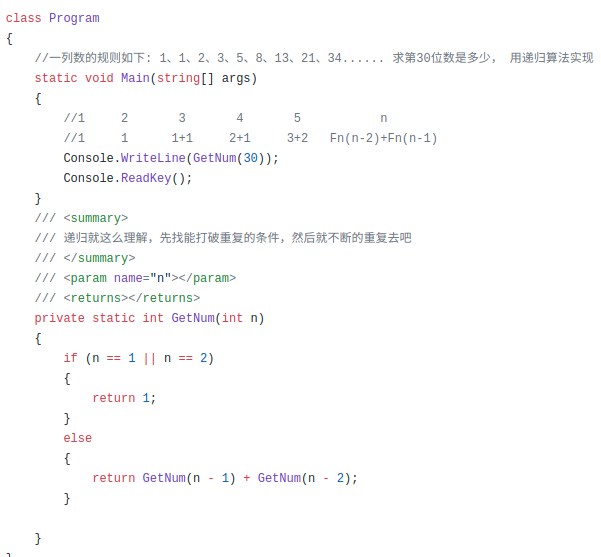2.匿名函数系列：lambda 参数: 表达式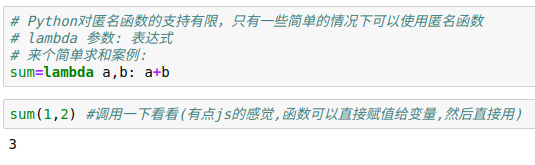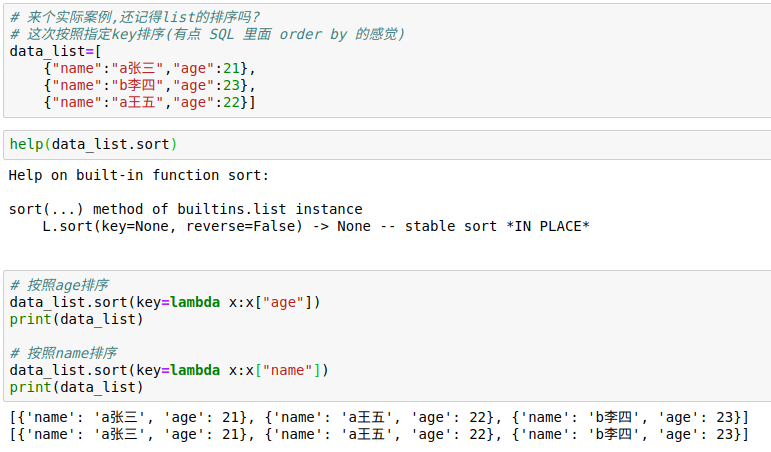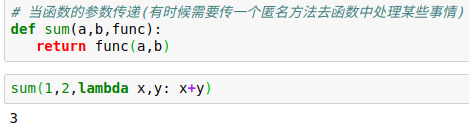NetCore：上面Code中的简写都是用匿名函数的方式，可以参考

3.全局变量和局部变量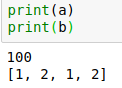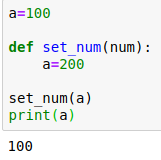# 这是因为，python定义一个变量的写法不用加类型导致的(⊙﹏⊙) 所以函数里面a=200，python解释器就认为你定义了一个和a这个全局变量名字相同的局部变量，那怎么用呢？global来声明一下全局变量即可：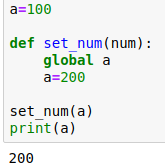# 在函数中不使用global声明全局变量不能修改全局变量==>本质是不能修改全局变量的指向，即不能将全局变量指向新的数据
# 对于不可变类型的全局变量来说，因其指向的数据不能修改，所以不使用global时无法修改全局变量
# 对于可变类型的全局变量来说，因其指向的数据可以修改，所以不使用global时也可修改全局变量

# 之前很多资料说py3匿名函数里不能调用print函数,自己测试下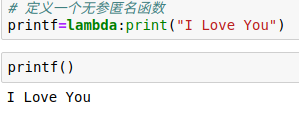# 可变类型与不可变类型的变量分别作为函数参数的区别
# 感到疑惑可以看之前的运算符扩展（https://www.cnblogs.com/dotnetcrazy/p/9155310.html#ext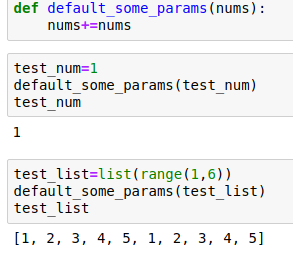# Python中函数参数都是引用传递
# 对于不可变类型，因变量不能修改，所以运算不会影响到变量自身
# 而对于可变类型来说，函数体中的运算有可能会更改传入的参数变量

# 函数名能不能重复的问题（能不能重载：具有不同的参数的类型或参数的个数【跟返回值没关系】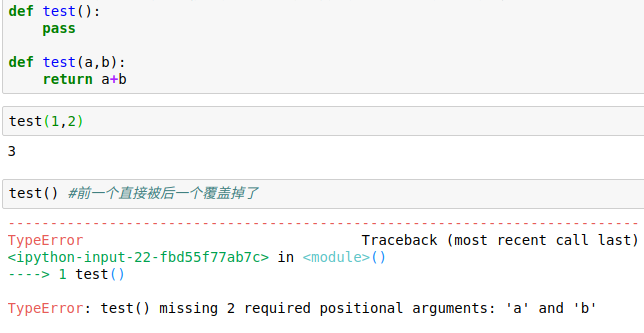1.交换两个数熟悉吧，编程第一课，除了用第三变量 或者 两数相加减 实现外，Python还有一种新方式a,b=b,a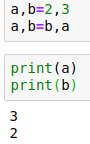NetCore:这个我也是刚知道，O(∩_∩)O哈哈~还得谢谢评论区的兄弟^_^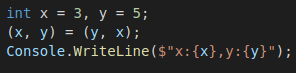2.eval（和js里面的eval差不多）：不太建议使用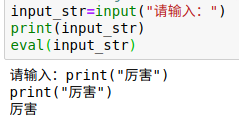posted @ 2018-06-15 11:28  毒逆天  阅读(1014)  评论(1编辑  收藏